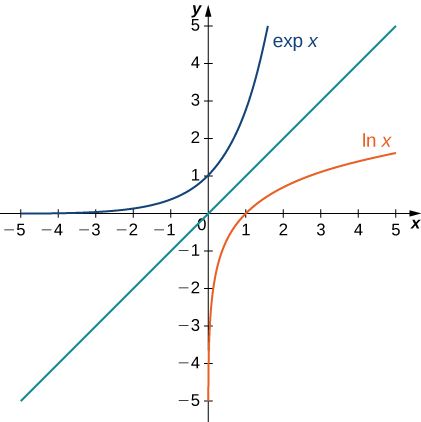# 6.7 Integrals, exponential functions, and logarithms  (Page 3/4)

 Page 3 / 4
$\text{exp}\left(\text{ln}\phantom{\rule{0.2em}{0ex}}x\right)=x\phantom{\rule{0.2em}{0ex}}\text{for}\phantom{\rule{0.2em}{0ex}}x>0\phantom{\rule{0.2em}{0ex}}\text{and}\phantom{\rule{0.2em}{0ex}}\text{ln}\left(\text{exp}\phantom{\rule{0.2em}{0ex}}x\right)=x\phantom{\rule{0.2em}{0ex}}\text{for all}\phantom{\rule{0.2em}{0ex}}x.$

The following figure shows the graphs of $\text{exp}\phantom{\rule{0.2em}{0ex}}x$ and $\text{ln}\phantom{\rule{0.2em}{0ex}}x.$The graphs of ln x and exp x .

We hypothesize that $\text{exp}\phantom{\rule{0.2em}{0ex}}x={e}^{x}.$ For rational values of $x,$ this is easy to show. If $x$ is rational, then we have $\text{ln}\left({e}^{x}\right)=x\phantom{\rule{0.2em}{0ex}}\text{ln}\phantom{\rule{0.2em}{0ex}}e=x.$ Thus, when $x$ is rational, ${e}^{x}=\text{exp}\phantom{\rule{0.2em}{0ex}}x.$ For irrational values of $x,$ we simply define ${e}^{x}$ as the inverse function of $\text{ln}\phantom{\rule{0.2em}{0ex}}x.$

## Definition

For any real number $x,$ define $y={e}^{x}$ to be the number for which

$\text{ln}\phantom{\rule{0.2em}{0ex}}y=\text{ln}\left({e}^{x}\right)=x.$

Then we have ${e}^{x}=\text{exp}\left(x\right)$ for all $x,$ and thus

${e}^{\text{ln}\phantom{\rule{0.2em}{0ex}}x}=x\phantom{\rule{0.2em}{0ex}}\text{for}\phantom{\rule{0.2em}{0ex}}x>0\phantom{\rule{0.2em}{0ex}}\text{and}\phantom{\rule{0.2em}{0ex}}\text{ln}\left({e}^{x}\right)=x$

for all $x.$

## Properties of the exponential function

Since the exponential function was defined in terms of an inverse function, and not in terms of a power of $e,$ we must verify that the usual laws of exponents hold for the function ${e}^{x}.$

## Properties of the exponential function

If $p$ and $q$ are any real numbers and $r$ is a rational number, then

1. ${e}^{p}{e}^{q}={e}^{p+q}$
2. $\frac{{e}^{p}}{{e}^{q}}={e}^{p-q}$
3. ${\left({e}^{p}\right)}^{r}={e}^{pr}$

## Proof

Note that if $p$ and $q$ are rational, the properties hold. However, if $p$ or $q$ are irrational, we must apply the inverse function definition of ${e}^{x}$ and verify the properties. Only the first property is verified here; the other two are left to you. We have

$\text{ln}\left({e}^{p}{e}^{q}\right)=\text{ln}\left({e}^{p}\right)+\text{ln}\left({e}^{q}\right)=p+q=\text{ln}\left({e}^{p+q}\right).$

Since $\text{ln}\phantom{\rule{0.2em}{0ex}}x$ is one-to-one, then

${e}^{p}{e}^{q}={e}^{p+q}.$

As with part iv. of the logarithm properties, we can extend property iii. to irrational values of $r,$ and we do so by the end of the section.

We also want to verify the differentiation formula for the function $y={e}^{x}.$ To do this, we need to use implicit differentiation. Let $y={e}^{x}.$ Then

$\begin{array}{ccc}\hfill \text{ln}\phantom{\rule{0.2em}{0ex}}y& =\hfill & x\hfill \\ \hfill \frac{d}{dx}\text{ln}\phantom{\rule{0.2em}{0ex}}y& =\hfill & \frac{d}{dx}x\hfill \\ \hfill \frac{1}{y}\phantom{\rule{0.1em}{0ex}}\frac{dy}{dx}& =\hfill & 1\hfill \\ \hfill \frac{dy}{dx}& =\hfill & y.\hfill \end{array}$

Thus, we see

$\frac{d}{dx}{e}^{x}={e}^{x}$

as desired, which leads immediately to the integration formula

$\int {e}^{x}dx={e}^{x}+C.$

We apply these formulas in the following examples.

## Using properties of exponential functions

Evaluate the following derivatives:

1. $\frac{d}{dt}{e}^{3t}{e}^{{t}^{2}}$
2. $\frac{d}{dx}{e}^{3{x}^{2}}$

We apply the chain rule as necessary.

1. $\frac{d}{dt}{e}^{3t}{e}^{{t}^{2}}=\frac{d}{dt}{e}^{3t+{t}^{2}}={e}^{3t+{t}^{2}}\left(3+2t\right)$
2. $\frac{d}{dx}{e}^{3{x}^{2}}={e}^{3{x}^{2}}6x$

Evaluate the following derivatives:

1. $\frac{d}{dx}\left(\frac{{e}^{{x}^{2}}}{{e}^{5x}}\right)$
2. $\frac{d}{dt}{\left({e}^{2t}\right)}^{3}$
1. $\frac{d}{dx}\left(\frac{{e}^{{x}^{2}}}{{e}^{5x}}\right)={e}^{{x}^{2}-5x}\left(2x-5\right)$
2. $\frac{d}{dt}{\left({e}^{2t}\right)}^{3}=6{e}^{6t}$

## Using properties of exponential functions

Evaluate the following integral: $\int 2x{e}^{\text{−}{x}^{2}}dx.$

Using $u$ -substitution, let $u=\text{−}{x}^{2}.$ Then $du=-2x\phantom{\rule{0.2em}{0ex}}dx,$ and we have

$\int 2x{e}^{\text{−}{x}^{2}}dx=\text{−}\int {e}^{u}du=\text{−}{e}^{u}+C=\text{−}{e}^{\text{−}{x}^{2}}+C.$

Evaluate the following integral: $\int \frac{4}{{e}^{3x}}dx.$

$\int \frac{4}{{e}^{3x}}dx=-\frac{4}{3}{e}^{-3x}+C$

## General logarithmic and exponential functions

We close this section by looking at exponential functions and logarithms with bases other than $e.$ Exponential functions are functions of the form $f\left(x\right)={a}^{x}.$ Note that unless $a=e,$ we still do not have a mathematically rigorous definition of these functions for irrational exponents. Let’s rectify that here by defining the function $f\left(x\right)={a}^{x}$ in terms of the exponential function ${e}^{x}.$ We then examine logarithms with bases other than $e$ as inverse functions of exponential functions.

## Definition

For any $a>0,$ and for any real number $x,$ define $y={a}^{x}$ as follows:

$y={a}^{x}={e}^{x\phantom{\rule{0.2em}{0ex}}\text{ln}\phantom{\rule{0.2em}{0ex}}a}.$

Now ${a}^{x}$ is defined rigorously for all values of x . This definition also allows us to generalize property iv. of logarithms and property iii. of exponential functions to apply to both rational and irrational values of $r.$ It is straightforward to show that properties of exponents hold for general exponential functions defined in this way.

#### Questions & Answers

Find the derivative of g(x)=−3.
Abdullah Reply
any genius online ? I need help!!
Guzorochi Reply
how can i help you?
Pina
need to learn polynomial
Zakariya
i will teach...
nandu
I'm waiting
Zakariya
plz help me in question
Abish
evaluate the following computation (x³-8/x-2)
Murtala Reply
teach me how to solve the first law of calculus.
Uncle Reply
what is differentiation
Ibrahim Reply
f(x) = x-2 g(x) = 3x + 5 fog(x)? f(x)/g(x)
Naufal Reply
fog(x)= f(g(x)) = x-2 = 3x+5-2 = 3x+3 f(x)/g(x)= x-2/3x+5
diron
pweding paturo nsa calculus?
jimmy
how to use fundamental theorem to solve exponential
JULIA Reply
find the bounded area of the parabola y^2=4x and y=16x
Omar Reply
what is absolute value means?
Geo Reply
Chicken nuggets
Hugh
🐔
MM
🐔🦃 nuggets
MM
(mathematics) For a complex number a+bi, the principal square root of the sum of the squares of its real and imaginary parts, √a2+b2 . Denoted by | |. The absolute value |x| of a real number x is √x2 , which is equal to x if x is non-negative, and −x if x is negative.
Ismael
find integration of loge x
Game Reply
find the volume of a solid about the y-axis, x=0, x=1, y=0, y=7+x^3
Godwin Reply
how does this work
Brad Reply
Can calculus give the answers as same as other methods give in basic classes while solving the numericals?
Cosmos Reply
log tan (x/4+x/2)
Rohan
please answer
Rohan
y=(x^2 + 3x).(eipix)
Claudia
is this a answer
Ismael
A Function F(X)=Sinx+cosx is odd or even?
WIZARD Reply
neither
David
Neither
Lovuyiso
f(x)=1/1+x^2 |=[-3,1]
Yuliana Reply
apa itu?
fauzi

### Read also:

#### Get Jobilize Job Search Mobile App in your pocket Now!

Source:  OpenStax, Calculus volume 1. OpenStax CNX. Feb 05, 2016 Download for free at http://cnx.org/content/col11964/1.2
Google Play and the Google Play logo are trademarks of Google Inc.

Notification Switch

Would you like to follow the 'Calculus volume 1' conversation and receive update notifications?By Wey HeyBy Marriyam RanaBy Jazzycazz JacksonBy OpenStaxBy Monty HartfieldBy Kimberly NicholsBy John GabrieliBy OpenStaxBy OpenStaxBy Sebastian Sieczko...